Conductors & Insulators

# Conductors & Insulators - Notes | Study Electromagnetic Fields Theory (EMFT) - Electrical Engineering (EE)

 1 Crore+ students have signed up on EduRev. Have you?

Self Energy of a Charge Distribution :

In Lecture 10 we briefly discussed what we called as the “Self energy problem”. We had seen that while the energy of a continuous charge distribution is positive, for a collection of discrete charges its sign could go either way.

The reason for this apparent anomaly was that when we considered a collection of point charges, it was implicitly assumed that no work is done in creating the point charges themselves; they were given to us, a priori. Suppose we are to assume that the discrete charges are essentially charges distributed over a sphere of radius R, calculate its energy a finally take the limit R → 0, the energy would turn out to be infinite! The energy of the electric field due to the charge distribution is given by W =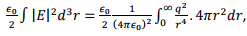which diverges in the lower limit.
Let us look at the interaction energy of two point charges, given by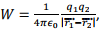using the expressions that we had derived for the continuous charge distribution, viz.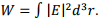Now the electric field due to the two charges obey the superposition principle, and is given by the sum of the fields due to the two charges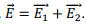However, when we substitute this in the expression for the energy, we have,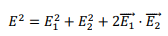Thus there is now an interference terms.

If we take the contribution to the energy from the first two terms, viz., from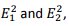each one of the terms will be infinite, since,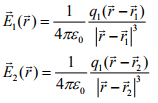Since the square of the fields go as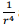and the volume element throws in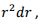the integrals diverge in the lower limit. These are the “self energy terms” which we neglect in our calculation of the interaction energy. The reason for such neglect is that experimentally we only measure the difference in energy with reference to the self energy. Self energy problem is not fully understood and remains a mathematical prescription. Let us consider the interaction energy term, given by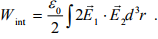Using the expressions for the electric fields given above, we can write the interaction energy as,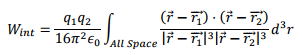This integral is not easy to perform but we will try to get as much information from it as possible.

Let us define,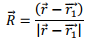In terms of which, we have,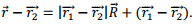In terms of this new variable, the interaction energy can be written as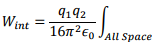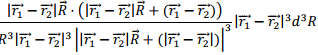Define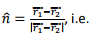a unit vector along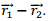We can express the above integral as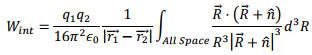We can rewrite the integrand, using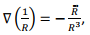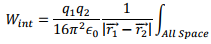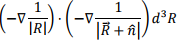We will simplify the integral using the following identity,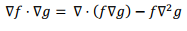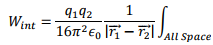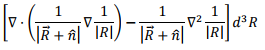The first term can be converted to a surface integral, using the divergence theorem and as before since the surface is at infinite distance, this term drops off. We are then left with the second term. Using the fact that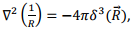this integral is readily performed and we get, as expected.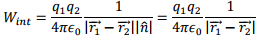Note that in going from the discrete charges to the case of continuous distribution of charges, we did so by providing a prescription,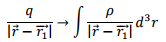However, in doing so, we did not include the fact that the “self interaction term”, i.e. the term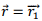term should have been excluded from the right hand side. As a result the divergence which should have been explicitly excluded have not been provided for.

But then, we did our calculation for the electrostatic energy of a uniformly charged sphere and did not encounter any divergence! The reason for this is somewhat subtle. In our continuous prescription, our volume elements can be made as small as we desire and as long as the charge density does not contain delta functions, we can make the volume element negligibly small and for any finite charge density (such as the case of uniform charge distribution) the amount of charge included in such infinitesimal volume become vanishingly small and its contribution to the potential vanishes. Thus in case of continuous charge distribution the self energy problem does not appear. Thus we do not see any self energy effect. The self energy problem is not fully understood and even in quantum electrodynamics.

Properties of Conductors:

Conductors are substances which have free electrons which can move under the action of an electric field. The electrons are free in the sense that they belong to the crystal as a whole and not tied down (bound) to a particular atom or a molecule.

Insulators, or dielectrics, on the other hand, are those substances in which the electrons retain their identity of being bound to an atom or a molecule. When subjected to an electric field, the electrons do get displaced from their positions, but still remain bound to the parent atom.

In reality, most material, even those who are good conductors such as copper or silver, offer some resistance to the motion of electrons when an electric field is applied. We will, however, assume that the conductors refer to ideal conductor having infinite conductivity. Likewise, the term insulator would signify that they do not allow motion of electrons in the crystal.

At this time we are discussing electrostatics i.e. an equilibrium situation. In an equilibrium there cannot be an electric field inside a conductor, for if it were not so, the electrons, under the action of electric force would accelerate and there would not be an equilibrium. When an electric field is applied, the electrons respond to it in an interesting way, restoring equilibrium in a very short time, of the order of 10-16 seconds. When we apply an electric field, the electrons are pushed to an edge of the conductor, leading to a charge separation. This results in an “internal” field being created which annuls the effect of the external field.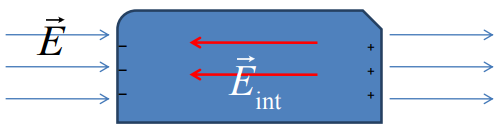Since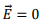inside a conductor, the divergence of the electric field, which is equal to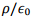is zero. In turn, this implies, that there cannot be a charge density inside a conductor. In any small volume, there would be equal positive and negative charges.

The charges in the conductor move its surface. Since the electric field inside is zero, the conductor is an equipotential,

Though there can be charges on the surface, the electric field cannot have a tangential component. If it did, the electrons on the surface would be accelerated, destroying equilibrium. The electric field outside the surface of a conductor can be found by taking a Gaussian surface, a cylinder, half of which is outside the surface and half inside. Suppose the cylinder passes through a small patch of area A on the surface. The charge enclosed by the cylinder is σA. Since the field is normal to the surface, only contribution to the flux is from the top face. As the field inside is zero, the flux is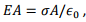which gives the field magnitude as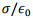Recall that we had seen that the field outside an infinite charged surface is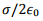This is because it had two faces contributing to The flux whereas here the field inside is zero.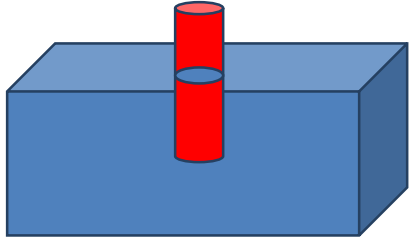A consequence of the field on the surface being normal to the surface is that the surface of a conductor is an equipotential. This is because we know that if we take any closed path in an electrostatic field, the line integral is zero. Consider a path on the surface connecting any two points A and B. We have,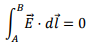because along the path the electric field is normal to the surface. However the line integral, by definition is the potential difference between B and A. Thus the conductor surface is an equipotential.

For a conductor of irregular shape, the charges tend to accumulate in region where the radius of curvature is the smallest, i.e., at sharp points. Consequently, the field in these regions will be the strongest. The assertion is somewhat difficult to prove rigorously but some qualitative idea is as follows.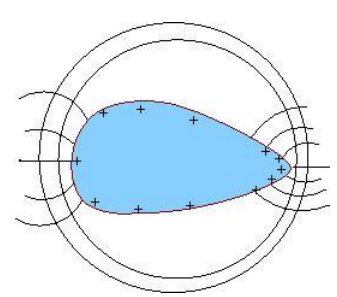From a long distance the charged conductor resembles a point charge and the equipotentials are spheres. Suppose we plot the equipotential surfaces such that successive surfaces have potential difference of v between them. As we come close to the conductor, the surfaces will not remain spherical but they cannot intersect. As we come closer, the surfaces will crowd up nearer the sharper edge. This implies that the electric field is the strongest in that region.

If there are charges in a conductor, they must reside only on the surface. This can be seen by application of Gauss’s law. If we take a Gaussian surface lying wholly within the material of the conductor (shown with green boundary), since the field is zero everywhere on the surface, it cannot enclose any charge. Thus any extra charge must move to the surface.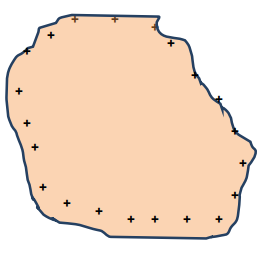Inside a cavity within a metal, there cannot be any charge on the cavity surface, i.e. charges, if any, must lie on the outside surface. The proof is very similar. Take a Gaussian surface lying entirely within the volume of the metal. This surface does not enclose any charge. Since there are no charges in the body of the metal, there cannot be any in the cavity surface either.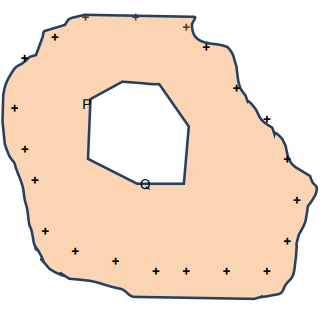Consider a contour which intersects the cavity at points P and Q and the rest of the contour lying within the body of the conductor (the red contour in the figure). We know that the integral of the electric field over any closed contour is zero. Further, the electric field itself being zero inside the volume of the conductor, the contribution of this part of the path is zero, leaving us with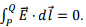However, the line integral is just the potential difference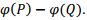Thus the points P and Q are at the same potential. Since P and Q are arbitrary points on the surface of the cavity and the path of integration lies inside the cavity, this is possible only if the field inside the cavity is zero everywhere.

This the principle of “Faraday Cage” which is used to isolate sensitive equipment from electrical disturbances. An equipment is shielded from external disturbances by enclosing it within a metal cage.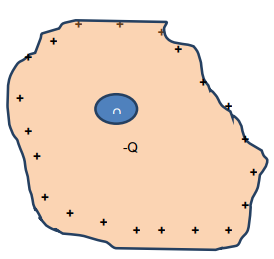Suppose by some means we have put a charge +Q inside the cavity. This can be done by using an insulating handle so that the charges remain within the cavity. Once again, using the Gaussian surface which encloses the cavity, but lies wholly within the bulk of the metal, the total charge enclosed is zero. Since there exists +Q charge within the cavity an equal and opposite charge – Q must appear on the inside surface of the cavity. Since the conductor itself is charge neutral, this can happen if a charge +Q appears on the outside surface of the conductor itself.

Pressure on a charged surface:

Consider a charged surface having a charge density σ. It turns out that the surface experiences a force, i.e. there is a pressure on any surface patch dS. If the electric field acting on the surface element is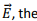force acting on this surface is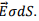This statement is somewhat ambiguous because we have seen earlier on that the electric field itself is discontinuous on the surface of a conductor. So which field does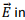this relation refer to? Is it the field above or below the patch dS?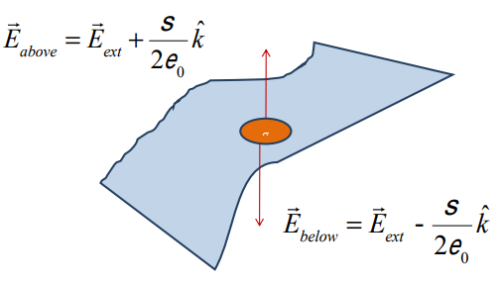Consider the small patch dS on the surface. If dS is taken sufficiently small, it can be considered as a flat area. Let this be taken to be in the xy plane so that the normal to the patch is in the z direction. In order to calculate the force on this patch let us mentally divide the entire surface into two parts, one part is dS itself and the other part is rest of the surface. The rest of the surface is essentially the original surface with a hole in place of the patch dS. Let us consider two points A and B, the former just above the patch and the latter just below the patch. The field at A can be considered due to two parts, one due to the charged surface with a hole and the other due to the patch itself. Since the surface with a hole is external to the patch, let us call the field due to it as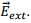The field at B due to this part is also the same, as A and B are very close to each other. There is no discontinuity for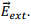Now let us look at the field due to the patch at A and B. This has a discontinuity. Let the field above be denoted as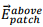and that below be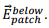These two fields are directed in opposite directions, the former above the patch and the latter below the patch. The magnitude of the field is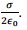The net fields above and below can be expressed as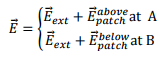Up to this point, the discussion is valid for all charged surfaces, not necessarily for a metal.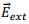can be seen to be given by the average of the fields at A and B,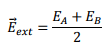If we consider a metal, the point B being within the conductor, the net field is zero, so that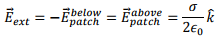Let us look at the force on the patch. The patch cannot exert force on itself. The force on the patch is due to the part of the surface other than the patch, i.e. due to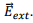The force on the patch is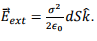This is the origin of the electrostatic pressure on a charged surface which is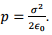In terms of the field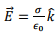outside the surface (i.e. at A) , it can be written as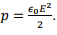The document Conductors & Insulators - Notes | Study Electromagnetic Fields Theory (EMFT) - Electrical Engineering (EE) is a part of the Electrical Engineering (EE) Course Electromagnetic Fields Theory (EMFT).
All you need of Electrical Engineering (EE) at this link: Electrical Engineering (EE)

## Electromagnetic Fields Theory (EMFT)

11 videos|46 docs|62 tests
 Use Code STAYHOME200 and get INR 200 additional OFF

## Electromagnetic Fields Theory (EMFT)

11 videos|46 docs|62 tests

Track your progress, build streaks, highlight & save important lessons and more!

,

,

,

,

,

,

,

,

,

,

,

,

,

,

,

,

,

,

,

,

,

;